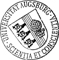## Biproportional Matrix Scaling and the Iterative Proportional Fitting Procedure

• Convergence of the Iterative Proportional Fitting procedure is analyzed. The input comprises a nonnegative weight matrix, and positive target marginals for rows and columns. The output sought is what is called the biproportional fit, a scaling of the input weight matrix by means of row and column divisors so as to equate row and column sums to target marginals. The procedure alternates between the fitting of rows, and the fitting of columns. We monitor progress with an L1-error function measuring the distance between current row and column sums and target row and column marginals. The procedure converges to the biproportional fit if and only if the L1-error tends zero. In case of non-convergence the procedure appears to oscillate between two accumulation points. The oscillation result is contingent on the "IPF conjecture" that row and column divisors are always convergent. The conjecture is established in the specific case when the even-step subsequence admits an accumulation pointConvergence of the Iterative Proportional Fitting procedure is analyzed. The input comprises a nonnegative weight matrix, and positive target marginals for rows and columns. The output sought is what is called the biproportional fit, a scaling of the input weight matrix by means of row and column divisors so as to equate row and column sums to target marginals. The procedure alternates between the fitting of rows, and the fitting of columns. We monitor progress with an L1-error function measuring the distance between current row and column sums and target row and column marginals. The procedure converges to the biproportional fit if and only if the L1-error tends zero. In case of non-convergence the procedure appears to oscillate between two accumulation points. The oscillation result is contingent on the "IPF conjecture" that row and column divisors are always convergent. The conjecture is established in the specific case when the even-step subsequence admits an accumulation point that is connected, but remains open in general.Author: Friedrich PukelsheimGND urn:nbn:de:bvb:384-opus4-23062 https://opus.bibliothek.uni-augsburg.de/opus4/2306 Preprints des Instituts für Mathematik der Universität Augsburg (2013-07) Preprint English Universität Augsburg 2013/04/16 alternating scaling algorithm; biproportional fitting; entropy; matrix scaling; RAS procedure Kontingenztafelanalyse; L1-Approximation; Statistik Mathematisch-Naturwissenschaftlich-Technische Fakultät Mathematisch-Naturwissenschaftlich-Technische Fakultät / Institut für Mathematik Mathematisch-Naturwissenschaftlich-Technische Fakultät / Institut für Mathematik / Lehrstuhl für Stochastik und ihre Anwendungen 5 Naturwissenschaften und Mathematik / 51 Mathematik / 510 MathematikDeutsches Urheberrecht mit Print on Demand